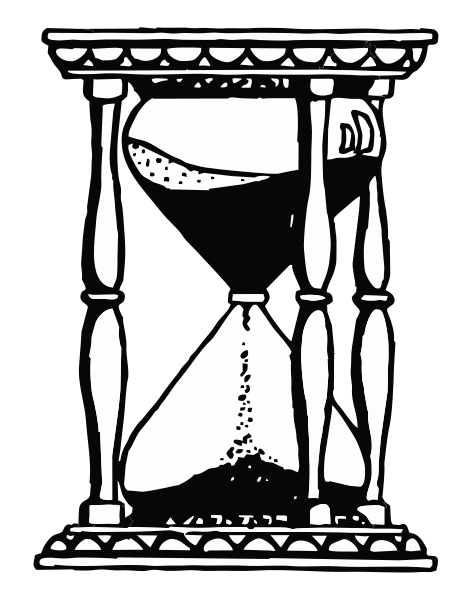## Rate-Determining Steps

#### Learning Objective

• Describe the relationship between the rate determining step and the rate law for chemical reactions

#### Key Points

• Chemists often write chemical equations for reactions as a single step, which shows only a reaction’s net result. However, most chemical reactions occur over a series of elementary reactions. The reaction mechanism is the step-by-step process by which reactants actually become products.
• The overall reaction rate depends almost entirely on the rate of the slowest step. If the first step is the slowest, and the entire reaction must wait for it, then it is the rate-determining step.

#### Term

• rate-determining stepThe slowest individual transformation in a reaction mechanism.

## Rate-Determining Steps

Chemists often write chemical equations for reactions as a single step that shows only the net result of a reaction. However, most chemical reactions occur over a series of elementary reactions. The complete sequence of these elementary steps is called a reaction mechanism. The reaction mechanism is the step-by-step process by which reactants actually become products. It is the “how” of the reaction, whereas the overall balanced equation shows only the “what” of the reaction. In kinetics, the rate of a reaction with several steps is determined by the slowest step, which is known as the rate-determining, or rate-limiting, step.

## Rate Laws and the Rate-Determining Step

Take the following example of a gas phase reaction:

$CO + NO_2 \rightarrow CO_2 + NO$

If this reaction occurred in a single step, its rate law would be:

$r = k[NO_2][CO]$

However, experiments show that the rate equation is:

$r = k[NO_2]^2$

The fact that the experimentally-determined rate law does not match the rate law derived from the overall reaction equation suggests that the reaction occurs over multiple steps. Further, the experimental rate law is second-order, suggesting that the reaction rate is determined by a step in which two NO2molecules react, and therefore the CO molecule must enter at another, faster step. A possible mechanism that explains the rate equation is:

$2\;NO_2 \rightarrow NO_3 + NO$ (slow step, rate-determining)

$NO_3 + CO \rightarrow NO_2 + CO_2$ (fast step)

Since the first step is the slowest, and the entire reaction must wait for it, it is the rate-determining step. We can picture the rate-determining step to be like the narrowest point in an hourglass; it is the “bottleneck” point of the reaction that determines how quickly reactants can become products.Hourglass analogy to the rate-determining stepThe rate-determining step is like the narrowest point in the hourglass; it is the “bottleneck” point of the reaction that determines how quickly reactants can become products.

If the first step in a mechanism is rate-determining, it is easy to find the rate law for the overall expression from the mechanism. If the second or a later step is rate-determining, determining the rate law is slightly more complicated. We will explore how to write that rate law later.